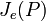# ZJe-subgroup

(diff) ← Older revision | Latest revision (diff) | Newer revision → (diff)
Let$p$ be a prime number and$P$ be a finite p-group. The ZJe-subgroup of$P$, denoted$Z(J_e(P))$, is defined as the center of the join of elementary abelian subgroups of maximum order (denoted$J_e(P)$). It is related to, but not necessarily the same as, the ZJ-subgroup, which is the center of the join of abelian subgroups of maximum order (denoted$J(P)$).$J_e(P)$ and$J(P)$ are two of the Thompson subgroups.